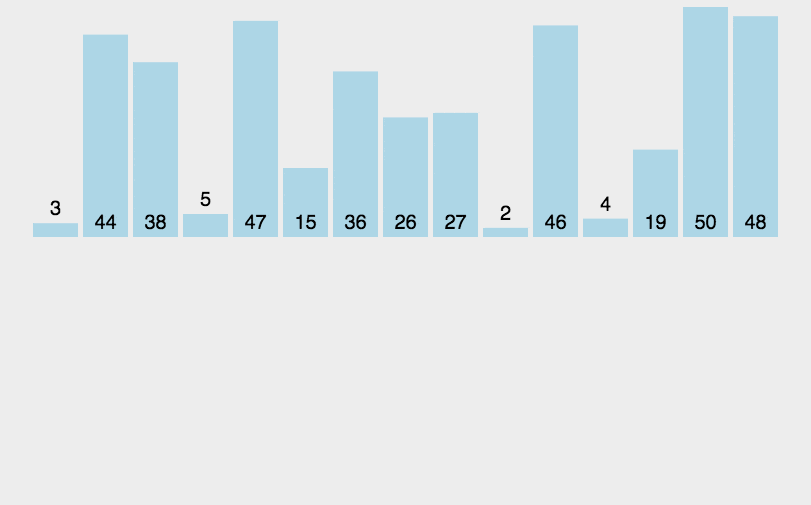## 稳定的直接插入排序# 时间复杂度分析## 最坏情况

$$\frac{n}{2^i}=1$$

## 平均时间复杂度

$$A(n)= \frac{1}{2n+1}(\sum_{i=1}^{k}i2^{i-1} + k(n+1))$$

$$\sum_{i=1}^{k}i2^{i-1} = 2^k(k-1)+1$$

$$\sum_{i=1}^{k}i2^{i-1}=0\times2^1+1+1\times2^2-0\times2^1+2\times2^3-1\times2^2+…+(k-1)2^k-(k-2)2^{k-1}=2^k(k-1)+1$$

$$A(n)= \frac{1}{2n+1}(\sum_{i=1}^{k}i2^{i-1} + k(n+1))$$
$$\sum_{i=1}^{k}i2^{i-1} = 2^k(k-1)+1$$

$$A(n) \approx \frac{1}{2^{k+1}}((k-1)2^{k}+k2^k)=\frac{(k-1)}{2}+\frac{k}{2}=k-\frac{1}{2}$$

# 代码实现# A+B for Input-Output Practice(using C++)

## 1.

Problem Description
Your task is to Calculate a + b. Too easy?! Of course! I specially designed the problem for acm beginners. You must have found that some problems have the same titles with this one, yes, all these problems were designed for the same aim
Input
The input will consist of a series of pairs of integers a and b, separated by a space, one pair of integers per line.
Output
For each pair of input integers a and b you should output the sum of a and b in one line, and with one line of output for each line in input.
Sample Input
1 6
1 20
Sample Output
7
21